### Home > PC > Chapter 2 > Lesson 2.2.2 > Problem2-62

2-62.
1. If the rational expressions have a common factor, it is usually easier to add the terms using the standard method of first finding a common denominator. Find the sums of the following. First find equivalent fractions as shown in the example below. Homework Help ✎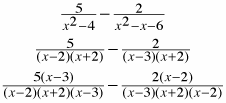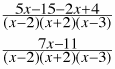1.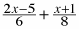2.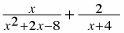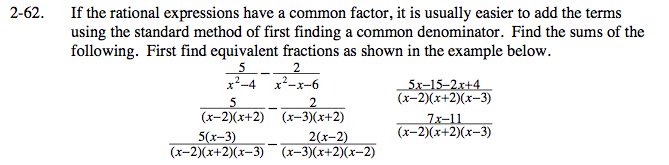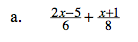$\frac{11x-17}{24}$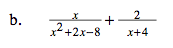$\frac{3x-4}{(x+4)(\textit{x}-2)}$